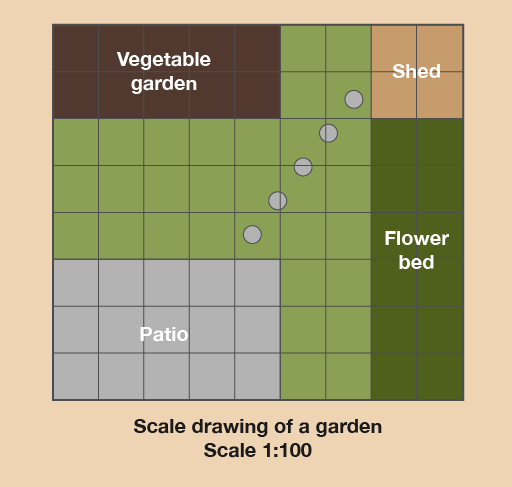Science, Maths & Technology

### Become an OU studentEveryday maths 1 (Wales)

Start this free course now. Just create an account and sign in. Enrol and complete the course for a free statement of participation or digital badge if available.

# 6 Scale drawings

Have you ever drawn a plan of a room in your house to help you work out how to rearrange the furniture? Or maybe you’ve sketched a plan of your garden to help you decide how big a new patio should be?

These pictures are called scale drawings. The important thing with scale drawings is that everything must be drawn to scale, meaning that everything must be in proportion – that is, ‘shrunk’ by the same amount.

All scale drawings must have a scale to tell us how much the drawing has been shrunk by.

## Example: In the garden

Here is an example of typical scale drawing:Figure 28 A scale drawing of a garden

What’s the width and length of the patio?

Hint: This scale drawing has been drawn on squared paper. This makes it easier to draw and understand. Each square is 1 cm wide and 1 cm long. So instead of using a ruler you can just count the squares and this will tell you the measurement in centimetres.

### Method

The scale in this drawing is 1:100. This means that 1 cm on the scale drawing is equal to 100 cm, or 1 m, in real life. Once we know the scale, we can measure the distances on the drawing.

Using a ruler (or just counting the squares), we find that the patio is 5 cm long and 3 cm wide on the drawing. This means that in real life it is 5 metres long and 3 metres wide.

So when you’re working with scale drawings:

• Find out what the scale on the drawing is.
• Measure the distance on the drawing using a ruler (or count the number of squares, if that’s an option). The measurements may already be given on the drawing.
• Multiply the distance you measure by the scale to give the distance in real life.
• If you already know the real-life measurement and need to work out the measurement on the drawing, you divide by the scale.

Now try the following activity. Remember to check your answers once you have completed the questions.

## Activity 12: Getting information from a scale drawing

1. Let’s stay with this scale drawing of the garden.Figure 29 A scale drawing of a garden
• a.What is the actual width and length of the vegetable garden?
• b.What is the actual width and length of the flower bed?
• c.How far is the patio from the vegetable garden in real life?
• d.Say you wanted to put a trampoline between the patio and the vegetable garden. It measures 3 m by 3 m in real life. Is there enough space for it?
2. Tom is using a scale drawing to plan out a patio he is going to lay. He uses a scale of 1 cm : 50 cm. The patio on his drawing measures 4 cm by 8 cm. What are the dimensions of his actual patio?
3. Amanda is drawing a plan of the ground floor of her house, using a scale of 1 cm: 2 m. Her actual kitchen measures 5 m by 6 m. What will the measurements be for her kitchen on the plan?

1. The answers are as follows:
• a.The vegetable garden is 5 m long and 2 m wide.
• b.The flower bed is 6 m long and 2 m wide.
• c.The patio and vegetable garden are 3 m apart.
• d.The distance between the patio and vegetable garden is 3 m and the trampoline is 3 m wide. So the trampoline would fit in the space, but it would be a bit of a squeeze.
2. The scale is 1 cm : 50 cm. There are two measurements to work out, so you need to do one at a time. We will start with the width of 4 cm:
• 1 cm : 50 cm
• 4 cm : ? cm
You know what the measurement on the drawing is, so you need to multiply to find the real-life measurement:
• 4 × 50 = 200 cm
You could then convert this measurement into metres. There are 100 cm in 1 metre, you need to divide by 100:
• 200 ÷ 100 = 2 m

So the width of the actual patio is 2 m.

We will now work out the length of the actual patio. The scale is the same:

• 1 cm : 50 cm
• 8 cm : ? cm
Again, you know the measurement on the drawing, so you need to multiply to find the real-life measurement:
• 8 × 50 = 400 cm
Again, we can divide this measurement by 100 to express it in metres:
• 400 ÷ 100 = 4 m
So the length of the actual patio is 4 m.
3. The scale is 1 cm : 2 m. There are two measurements to work out, so you need to do one at a time. We will start with the width of 5 m:
• 1 cm : 2 m
• ? cm : 5 m
As you know the real measurement, you need to divide to find the plan measurement:
• 5 ÷ 2 = 2.5 cm
We will now work out the length. The scale is the same:
• 1 cm : 2 m
• ? cm : 6 m
Again, we need to work out the plan measurement, so we need to divide:
• 6 ÷ 2 = 3 cm
So on the plan, her kitchen will measure 2.5 cm by 3 cm.

## Summary

In this section you have learned how to use scale drawings.